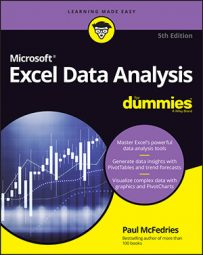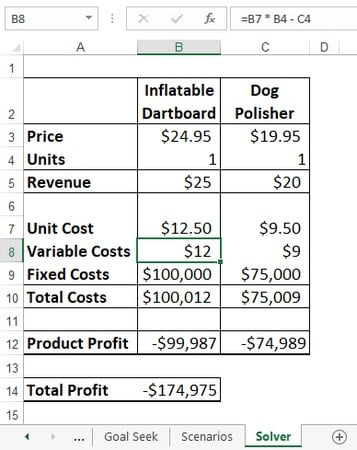##### Excel Data Analysis For DummiesExcel spreadsheet tools such as Goal Seek that change a single variable are useful, but unfortunately most problems in business are not so easy. You’ll usually face formulas with at least two and sometimes dozens of variables. Often, a problem will have more than one solution, and your challenge will be using Excel to find the optimal solution (that is, the one that maximizes profit, or minimizes costs, or matches other criteria). For these bigger challenges, you need a more muscular tool. Excel has just the answer: Solver. Excel Solver is a sophisticated optimization program that enables you to find the solutions to complex problems that would otherwise require high-level mathematical analysis.

## Understanding Excel Solver

Solver, like Goal Seek, uses an iterative method to perform its calculations. Using iteration means that Excel Solver tries a solution, analyzes the results, tries another solution, and so on. However, this cyclic iteration isn’t just guesswork on Solver’s part. That would be silly. No, Excel Solver examines how the results change with each new iteration and, through some sophisticated mathematical processes (which, thankfully, happen way in the background and can be ignored), can usually tell in what direction it should head for the solution.

## The advantages of the Excel Solver

Yes, Goal Seek and Solver are both iterative, but that doesn’t make them equal. In fact, Excel Solver brings a number of advantages to the table:
• Excel Solver enables you to specify multiple adjustable cells. You can use up to 200 adjustable cells in all.
• Excel Solver enables you to set up constraints on the adjustable cells. For example, you can tell Solver to find a solution that not only maximizes profit, but also satisfies certain conditions, such as achieving a gross margin between 20 and 30 percent, or keeping expenses less than \$100,000. These conditions are said to be constraints on the solution.
• Excel Solver seeks not only a desired result (the “goal” in Goal Seek) but also the optimal one. For example, looking for an optimal result might mean that you can find a solution that’s the maximum or minimum possible.
• For complex problems, Solver can generate multiple solutions. You can then save these different solutions under different scenarios.

## When should you use Excel Solver?

Okay, let’s shoot straight here: Excel Solver is a powerful tool that most Excel users don’t need. It would be overkill, for example, to use Solver to compute net profit given fixed revenue and cost figures. Many problems, however, require nothing less than the Solver approach. These problems cover many different fields and situations, but they all have the following characteristics in common:
• They have a single objective cell (also called the target cell) that contains a formula you want to maximize, minimize, or set to a specific value. This formula could be a calculation such as total transportation expenses or net profit.
• The objective cell formula contains references to one or more variable cells (also called unknowns or changing cells). Solver adjusts these cells to find the optimal solution for the objective cell formula. These variable cells might include items such as units sold, shipping costs, or advertising expenses.
• Optionally, there are one or more constraint cells that must satisfy certain criteria. For example, you might require that advertising be less than 10 percent of total expenses, or that the discount to customers be an amount between 40 and 60 percent.
For example, the image below shows a worksheet data model that’s all set up for Excel Solver. The model shows revenue (price times units sold) and costs for two products, the profit produced by each products, and the total profit. The question to be answered here is this: How many units of each product must be sold to get a total profit of \$0? This is known in business as a break-even analysis.The goal for this data model is to find the break-even point (where total profit is \$0).

That sounds like a straightforward Goal Seek task, but this model has a tricky aspect: the variable costs. Normally, the variable costs of a product are its unit cost times the number of units sold. If it costs \$10 to produce product A, and you sell 10,000 units, the variable costs for that product are \$100,000. However, in the real world, such costs are often mixed up among multiple products. For example, if you run a joint advertising campaign for two products, those costs are borne by both products. Therefore, this model assumes that the costs of one product are related to the units sold of the other. Here, for example, is the formula used to calculate the costs of the Inflatable Dartboard (cell B8):

`=B7 * B4 – C4`
In other words, the variable costs for the Inflatable Dartboard are reduced by one dollar for every unit sold of the Dog Polisher. The latter’s variable costs use a similar formula (in cell C8):
`=C7 * C4 – B4`
Having the variable costs related to multiple products puts this data model outside of what Goal Seek can do, but Solver is up to the challenge. Here are the special cells in the model that Solver will use:
• The objective cell is C14; the total profit and the target solution for this formula is 0 (that is, the break-even point).
• The changing cells are B4 and C4, which hold the number of units sold for each product.
• For constraints, you might want to add that both the product profit cells (B12 and C12) should also be 0.

An add-in is software that adds one or more features to Excel. Installing add-ins gives you additional Excel features that aren’t available in the Ribbon by default. Bundled add-in software is included with Excel but isn’t automatically installed when you install Excel. Several add-ins come standard with Excel, including Solver, which enables you to solve optimization problems.

You install the bundled add-ins by using the Excel Options dialog box; you can find them in the Add-Ins section. After they're installed, add-ins are available right away. They usually appear on a tab related to their function. For example, Solver appears on the Data tab.

1. Choose File→ Options.

The Excel Options dialog box appears.

3. In the Manage list, select Excel Add-Ins and then select Go.

Excel displays the Add-Ins dialog box.

4. Select the Solver Add-In check box
5. Click OK.

Excel adds a Solver button to the Data tab’s Analyze group.

Take a look to find out how to use the Excel Solver.